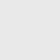# Decimal to Binary Converter

A important process in computer science and digital electronics is the translation of decimal to binary. The only two digits in the base-2 numeral system known as binary are 0 and 1. Decimal, on the other hand, uses ten digits, ranging from 0 to 9, and is a base-10 numeral system. Computing and circuit design both require the conversion of decimal numbers to binary.

## Decimal to Binary Converter ⬇️

In this article, we’ll explore the process of converting a decimal number to binary and provide an example of how to use this process to convert a decimal number to binary. We’ll also discuss the importance of decimal to binary conversion in computer science and digital electronics.

## Process of Decimal to Binary Conversion

The process of converting a decimal number to binary involves dividing the decimal number by 2 repeatedly and keeping track of the remainders. Here are the steps:

1. Divide the decimal number by 2.
2. Write down the quotient (the result of the division) and the remainder (either 0 or 1).
3. Repeat steps 1 and 2 with the quotient until the quotient becomes 0.
4. Write the remainders in reverse order to get the binary equivalent of the decimal number.

Below shown image is example of converting Decimal to Binary :

## Example of Decimal to Binary Conversion

Let’s use an example to illustrate this process. Let’s convert the decimal number 25 to binary.

1. Divide 25 by 2. The quotient is 12, and the remainder is 1. Write down “1” as the rightmost digit of the binary number.
2. Divide 12 by 2. The quotient is 6, and the remainder is 0. Write down “0” as the next digit of the binary number.
3. Divide 6 by 2. The quotient is 3, and the remainder is 0. Write down “0” as the next digit of the binary number.
4. Divide 3 by 2. The quotient is 1, and the remainder is 1. Write down “1” as the next digit of the binary number.
5. Since the quotient is 1, we stop dividing. The binary equivalent of 25 is 11001.

Here is another example of 244 conversion to binary:

## Importance of Decimal to Binary Conversion

Decimal to binary conversion is an essential operation in computer science and digital electronics. Computers and other digital devices use binary numbers to store and process information. Decimal to binary conversion is used to convert decimal numbers to binary so that computers and other digital devices can understand and process them.

Decimal to binary conversion is also used in digital electronics to design and build digital circuits. Digital circuits use binary signals (0 and 1) to represent information. Decimal to binary conversion is used to convert decimal numbers to binary signals that can be used by digital circuits.

## Conclusion

Decimal to binary conversion is a fundamental operation in computer science and digital electronics. It is used to convert decimal numbers to binary so that computers and other digital devices can understand and process them. Decimal to binary conversion is also used in digital electronics to design and build digital circuits. By following the simple process outlined in this article, you can easily convert decimal numbers to binary.

## Subscribe to our weekly newsletter

Subscribe
Notify ofInline Feedbacks

## Browse## Browse